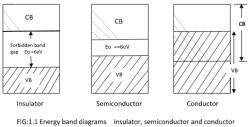Courses

# Test: PN Diode

## 10 Questions MCQ Test Physics For JEE | Test: PN Diode

Description
This mock test of Test: PN Diode for JEE helps you for every JEE entrance exam. This contains 10 Multiple Choice Questions for JEE Test: PN Diode (mcq) to study with solutions a complete question bank. The solved questions answers in this Test: PN Diode quiz give you a good mix of easy questions and tough questions. JEE students definitely take this Test: PN Diode exercise for a better result in the exam. You can find other Test: PN Diode extra questions, long questions & short questions for JEE on EduRev as well by searching above.
QUESTION: 1

### A photodiode is used preferably in

Solution:

Photo diode is used to detect light.
In a reverse biased condition, the width of the depletion region increases as the applied reverse bias voltage increases across the diode. So, by applying a larger voltage, more of the incident photons are converted to electric current thereby increasing the efficiency.
In forward biased conditions, the width of the depletion region decreases so only a small portion of the incident photons get converted to electric current and hence the efficiency is less.
Hence photo diode in reverse biased condition detects light.

QUESTION: 2

### When a forward bias is applied to a p-n junction, it

Solution:

When a forward bias is applied to a p-n junction it lowers the value of potential barrier. In the case of a forward bias the potential barrier opposes the applied voltage. Hence the potential barrier across the junction gets reduced.

QUESTION: 3

### Which of the following is true for a forward-biased diode?

Solution:

There are two types of ions -anions are the - ve ones & cations are the +ve ones.As we know current flow from +ve to - ve, so During electrolysis or forward biasing anions move to anodes that are +ve in nature whereas cations move to cathodes which are -ve in nature.

QUESTION: 4

The diode current depends on which of the following:

Solution:

The diode will reach its trigger point at lower voltage with rise in ambient temp, and at higher voltage for lower temp. As increased in temp, it accounts larger diode current than its previous current. When we talk about REVERSE SATURATION CURRENT, yes the diode current is dependable on it, because the (IS) i.e., reverse saturation current mainly depends on temp.

QUESTION: 5

The name of a diode that can be used to provide a variable capacitance is:

Solution:

The varactor diode is used in a place where the variable capacitance is required, and that capacitance is controlled with the help of the voltage. The Varactor diode is also known as the Varicap, Voltcap, Voltage variable capacitance or Tunning diode.

QUESTION: 6

Which of the statements is true for p-type semiconductors?

Solution:

In a p-type semiconductor, the holes are the majority carriers, while the electrons are the minority carriers. A p-type semiconductor is obtained when trivalent atoms, such as aluminium, are doped in silicon atoms.

QUESTION: 7

In an unbiased p-n junction, holes diffuse from the p-region to n-region because

Solution:

The diffusion of charge carriers across a junction takes place from the region of higher concentration to the region of lower concentration. In this case, the p-region has greater concentration of holes than the n-region. Hence, in an unbiased p-n junction, holes diffuse from the p-region to the n-region.

QUESTION: 8

Why does current flow in the forward-bias direction but not flow in the reverse-bias direction in a pn junction?

Solution:

The p-n junction has the very useful property that electrons are only able to flow in one direction. As current consists of a flow of electrons, this means that current is allowed to flow only in one direction across the structure, but it is stopped from flowing in the other direction across the junction.

QUESTION: 9

In an n- type silicondiode, which of the following statement is true :

Solution:

In an n-type silicon, the electrons are the majority carriers, while the holes are the minority carriers. An n-type semiconductor is obtained when pentavalent atoms, such as phosphorus, are doped in silicon atoms.

QUESTION: 10

P-type material is created by using a dopant from group:

Solution:

Atoms with one less valence electron result in "p-type" material. These p-type materials are group III elements in the periodic table. Therefore, p-type material has only 3 valence electrons with which to interact with silicon atoms.

Track your progress, build streaks, highlight & save important lessons and more!

### Similar Content### Related tests